### OUTPUT Statement

OUTPUT < OUT=SAS-data-set > output-options ;

The OUTPUT statement creates a SAS data set that contains statistics that are computed by PROC FREQ. Table 3.7 lists the statistics that can be stored in the output data set. You identify which statistics to include by specifying output-options.

You must use a TABLES statement with the OUTPUT statement. The OUTPUT statement stores statistics for only one table request. If you use multiple TABLES statements, the contents of the output data set correspond to the last TABLES statement. If you use multiple table requests in a single TABLES statement, the contents of the output data set correspond to the last table request. Only one OUTPUT statement is allowed in a single invocation of the procedure.

For a one-way or two-way table, the output data set contains one observation that stores the requested statistics for the table. For a multiway table, the output data set contains an observation for each two-way table (stratum) of the multiway crosstabulation. If you request summary statistics for the multiway table, the output data set also contains an observation that stores the across-strata summary statistics. If you use a BY statement, the output data set contains an observation or set of observations for each BY group. For more information about the contents of the output data set, see the section Contents of the OUTPUT Statement Output Data Set.

The output data set that is created by the OUTPUT statement is not the same as the output data set that is created by the OUT= option in the TABLES statement. The OUTPUT statement creates a data set that contains statistics (such as the Pearson chi-square and its p-value), and the OUT= option in the TABLES statement creates a data set that contains frequency table counts and percentages. See the section Output Data Sets for more information.

As an alternative to the OUTPUT statement, you can use the Output Delivery System (ODS) to store statistics that PROC FREQ computes. ODS can create a SAS data set from any table that PROC FREQ produces. See the section ODS Table Names for more information.

You can specify the following options in the OUTPUT statement:

OUT=SAS-data-set

specifies the name of the output data set. When you use an OUTPUT statement but do not use the OUT= option, PROC FREQ creates a data set and names it by using the DATAn convention.

output-options

specify the statistics to include in the output data set. Table 3.7 lists the output-options that are available in the OUTPUT statement, together with the TABLES statement options that are required to produce the statistics. Descriptions of the output-options follow the table in alphabetical order.

You can specify output-options to request individual statistics, or you can request groups of statistics by using output-options that are identical to the group options in the TABLES statement (for example, the CHISQ, MEASURES, CMH, AGREE, and ALL options).

When you specify an output-option, the output data set includes statistics from the corresponding analysis. In addition to the estimate or test statistic, the output data set includes associated values such as standard errors, confidence limits, p-values, and degrees of freedom. See the section Contents of the OUTPUT Statement Output Data Set for details.

To store a statistic in the output data set, you must also request computation of that statistic with the appropriate TABLES, EXACT, or TEST statement option. For example, the PCHI output-option includes the Pearson chi-square in the output data set. You must also request computation of the Pearson chi-square by specifying the CHISQ option in the TABLES statement. Or, if you use only one TABLES statement, you can request computation of the Pearson chi-square by specifying the PCHI or CHISQ option in the EXACT statement. Table 3.7 lists the TABLES statement options that are required to produce the OUTPUT data set statistics.

Table 3.7: OUTPUT Statement Output Options

Output Option

Output Data Set Statistics

Required TABLES Statement Option

McNemar’s test (tables), Bowker’s test,

simple and weighted kappas; for multiple strata,

overall simple and weighted kappas, tests for equal

kappas, and Cochran’s Q (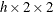tables)

Continuity-adjusted chi-square (tables)

CHISQ, MEASURES, and CMH statistics;

N (number of nonmissing observations)

Breslow-Day test (tables)

CMH, CMH1, or CMH2

Binomial statistics (one-way tables)

For one-way tables, goodness-of-fit test;

for two-way tables, Pearson, likelihood ratio,

chi-squares, Fisher’s exact test (tables),

phi and contingency coefficients, Cramér’s V

Cochran-Mantel-Haenszel (CMH) correlation,

row mean scores (ANOVA), and general

association statistics; fortables, logit

and Mantel-Haenszel common odds ratios

and relative risks, Breslow-Day test

CMH statistics, except row mean scores (ANOVA)

CMH or CMH1

and general association statistics

CMH statistics, except general association statistic

CMH or CMH2

CMH correlation statistic

CMH, CMH1, or CMH2

CMH general association statistic

CMH row mean scores (ANOVA) statistic

CMH or CMH2

Cochran’s Q (tables)

Contingency coefficient

Cramér’s V

Test for equal simple kappas

Zelen’s test for equal odds ratios (tables)

Test for equal weighted kappas

Fisher’s exact test

CHISQ or FISHER [a]

Gamma

Gail-Simon test

Jonckheere-Terpstra test

Simple kappa coefficient

Kendall’s tau-b

Lambda asymmetric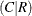Lambda symmetric

Lambda asymmetric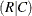Logit common odds ratio

CMH, CMH1, or CMH2

Logit common relative risk, column 1

CMH, CMH1, or CMH2

Logit common relative risk, column 2

CMH, CMH1, or CMH2

Likelihood ratio chi-square

McNemar’s test (tables)

Gamma, Kendall’s tau-b, Stuart’s tau-c,

Somers’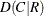and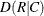, Pearson and

Spearman correlations, lambda asymmetricand, lambda symmetric,

uncertainty coefficientsand,

symmetric uncertainty coefficient;

odds ratio and relative risks (tables)

Mantel-Haenszel chi-square

Mantel-Haenszel common odds ratio

CMH, CMH1, or CMH2

Mantel-Haenszel common relative risk, column 1

CMH, CMH1, or CMH2

Mantel-Haenszel common relative risk, column 2

CMH, CMH1, or CMH2

Number of nonmissing observations

Number of missing observations

Odds ratio (tables)

Chi-square goodness-of-fit test (one-way tables),

Pearson chi-square (two-way tables)

Pearson correlation coefficient

Phi coefficient

Polychoric correlation coefficient

Column 1 risk difference (row 1 – row 2)

Column 2 risk difference (row 1 – row 2)

Odds ratio and relative risks (tables)

Risks and risk differences (tables)

Risks and risk difference, column 1

Risks and risk difference, column 2

Relative risk, column 1

Relative risk, column 2

Column 1 overall risk

Column 1 risk for row 1

Column 2 risk for row 1

Column 2 overall risk

Column 1 risk for row 2

Column 2 risk for row 2

Spearman correlation coefficient

Somers’Somers’Stuart’s tau-c

Cochran-Armitage test for trend

Bowker’s test of symmetry

Symmetric uncertainty coefficient

Uncertainty coefficientUncertainty coefficientWeighted kappa coefficient

[a] CHISQ computes Fisher’s exact test fortables. Use the FISHER option to compute Fisher’s exact test for generaltables.

You can specify the following output-options in the OUTPUT statement.

AGREE

includes the following tests and measures of agreement in the output data set: McNemar’s test (fortables), Bowker’s test of symmetry, the simple kappa coefficient, and the weighted kappa coefficient. For multiway tables, the AGREE option also includes the following statistics in the output data set: overall simple and weighted kappa coefficients, tests for equal simple and weighted kappa coefficients, and Cochran’s Q test.

The AGREE option in the TABLES statement requests computation of tests and measures of agreement. See the section Tests and Measures of Agreement for details about these statistics.

AGREE statistics are computed only for square tables, where the number of rows equals the number of columns. PROC FREQ provides Bowker’s test of symmetry and weighted kappa coefficients only for tables larger than. (Fortables, Bowker’s test is identical to McNemar’s test, and the weighted kappa coefficient equals the simple kappa coefficient.) Cochran’s Q is available for multiwaytables.

AJCHI

includes the continuity-adjusted chi-square in the output data set. The continuity-adjusted chi-square is available fortables and is provided by the CHISQ option in the TABLES statement. See the section Continuity-Adjusted Chi-Square Test for details.

ALL

includes all statistics that are requested by the CHISQ, MEASURES, and CMH output-options in the output data set. ALL also includes the number of nonmissing observations, which you can request individually by specifying the N output-option.

BDCHI

includes the Breslow-Day test in the output data set. The Breslow-Day test for homogeneity of odds ratios is computed for multiwaytables and is provided by the CMH, CMH1, and CMH2 options in the TABLES statement. See the section Breslow-Day Test for Homogeneity of the Odds Ratios for details.

BINOMIAL
BIN

includes the binomial proportion estimate, confidence limits, and tests in the output data set. The BINOMIAL option in the TABLES statement requests computation of binomial statistics, which are available for one-way tables. See the section Binomial Proportion for details.

CHISQ

includes the following chi-square tests and measures in the output data set for two-way tables: Pearson chi-square, likelihood ratio chi-square, Mantel-Haenszel chi-square, phi coefficient, contingency coefficient, and Cramér’s V. Fortables, CHISQ also includes Fisher’s exact test and the continuity-adjusted chi-square in the output data set. See the section Chi-Square Tests and Statistics for details. For one-way tables, CHISQ includes the chi-square goodness-of-fit test in the output data set. See the section Chi-Square Test for One-Way Tables for details. The CHISQ option in the TABLES statement requests computation of these statistics.

If you specify the CHISQ(WARN=OUTPUT) option in the TABLES statement, the CHISQ option also includes the variable WARN_PCHI in the output data set. This variable indicates the validity warning for the asymptotic Pearson chi-square test.

CMH

includes the following Cochran-Mantel-Haenszel statistics in the output data set: correlation, row mean scores (ANOVA), and general association. Fortables, the CMH option also includes the Mantel-Haenszel and logit estimates of the common odds ratio and relative risks. For multiway (stratified)tables, the CMH option includes the Breslow-Day test for homogeneity of odds ratios. The CMH option in the TABLES statement requests computation of these statistics. See the section Cochran-Mantel-Haenszel Statistics for details.

If you specify the CMH(MF) option in the TABLES statement, the CMH option includes the Mantel-Fleiss analysis in the output data set. The variables MF_CMH and WARN_CMH contain the Mantel-Fleiss criterion and the warning indicator, respectively.

CMH1

includes the CMH statistics in the output data set, with the exception of the row mean scores (ANOVA) statistic and the general association statistic. The CMH1 option in the TABLES statement requests computation of these statistics. See the section Cochran-Mantel-Haenszel Statistics for details.

CMH2

includes the CMH statistics in the output data set, with the exception of the general association statistic. The CMH2 option in the TABLES statement requests computation of these statistics. See the section Cochran-Mantel-Haenszel Statistics for details.

CMHCOR

includes the Cochran-Mantel-Haenszel correlation statistic in the output data set. The CMH option in the TABLES statement requests computation of this statistic. See the section Correlation Statistic for details.

CMHGA

includes the Cochran-Mantel-Haenszel general association statistic in the output data set. The CMH option in the TABLES statement requests computation of this statistic. See the section General Association Statistic for details.

CMHRMS

includes the Cochran-Mantel-Haenszel row mean scores (ANOVA) statistic in the output data set. The CMH option in the TABLES statement requests computation of this statistic. See the section ANOVA (Row Mean Scores) Statistic for details.

COCHQ

includes Cochran’s Q test in the output data set. The AGREE option in the TABLES statement requests computation of this test, which is available for multiwaytables. See the section Cochran’s Q Test for details.

CONTGY

includes the contingency coefficient in the output data set. The CHISQ option in the TABLES statement requests computation of the contingency coefficient. See the section Contingency Coefficient for details.

CRAMV

includes Cramér’s V in the output data set. The CHISQ option in the TABLES statement requests computation of Cramér’s V. See the section Cramér’s V for details.

EQKAP

includes the test for equal simple kappa coefficients in the output data set. The AGREE option in the TABLES statement requests computation of this test, which is available for multiway, square (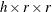) tables. See the section Tests for Equal Kappa Coefficients for details.

EQOR
ZELEN

includes Zelen’s exact test for equal odds ratios in the output data set. The EQOR option in the EXACT statement requests computation of this test, which is available for multiwaytables. See the section Zelen’s Exact Test for Equal Odds Ratios for details.

EQWKP

includes the test for equal weighted kappa coefficients in the output data set. The AGREE option in the TABLES statement requests computation of this test. The test for equal weighted kappas is available for multiway, square () tables where r > 2. See the section Tests for Equal Kappa Coefficients for details.

FISHER

includes Fisher’s exact test in the output data set. Fortables, the CHISQ option in the TABLES statement provides Fisher’s exact test. For tables larger than, the FISHER option in the EXACT statement provides Fisher’s exact test. See the section Fisher’s Exact Test for details.

GAMMA

includes the gamma statistic in the output data set. The MEASURES option in the TABLES statement requests computation of the gamma statistic. See the section Gamma for details.

GS
GAILSIMON

includes the Gail-Simon test for qualitative interaction in the output data set. The CMH(GAILSIMON) option in the TABLES statement requests computation of this test. See the section Gail-Simon Test for Qualitative Interactions for details.

JT

includes the Jonckheere-Terpstra test in the output data set. The JT option in the TABLES statement requests the Jonckheere-Terpstra test. See the section Jonckheere-Terpstra Test for details.

KAPPA

includes the simple kappa coefficient in the output data set. The AGREE option in the TABLES statement requests computation of kappa, which is available for square tables (where the number of rows equals the number of columns). For multiway square tables, the KAPPA option also includes the overall kappa coefficient in the output data set. See the sections Simple Kappa Coefficient and Overall Kappa Coefficient for details.

KENTB
TAUB

includes Kendall’s tau-b in the output data set. The MEASURES option in the TABLES statement requests computation of Kendall’s tau-b. See the section Kendall’s Tau-b for details.

LAMCR

includes the asymmetric lambda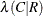in the output data set. The MEASURES option in the TABLES statement requests computation of lambda. See the section Lambda (Asymmetric) for details.

LAMDAS

includes the symmetric lambda in the output data set. The MEASURES option in the TABLES statement requests computation of lambda. See the section Lambda (Symmetric) for details.

LAMRC

includes the asymmetric lambda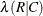in the output data set. The MEASURES option in the TABLES statement requests computation of lambda. See the section Lambda (Asymmetric) for details.

LGOR

includes the logit estimate of the common odds ratio in the output data set. The CMH option in the TABLES statement requests computation of this statistic, which is available fortables. See the section Adjusted Odds Ratio and Relative Risk Estimates for details.

LGRRC1

includes the logit estimate of the common relative risk (column 1) in the output data set. The CMH option in the TABLES statement requests computation of this statistic, which is available fortables. See the section Adjusted Odds Ratio and Relative Risk Estimates for details.

LGRRC2

includes the logit estimate of the common relative risk (column 2) in the output data set. The CMH option in the TABLES statement requests computation of this statistic, which is available fortables. See the section Adjusted Odds Ratio and Relative Risk Estimates for details.

LRCHI

includes the likelihood ratio chi-square in the output data set. The CHISQ option in the TABLES statement requests computation of the likelihood ratio chi-square. See the section Likelihood Ratio Chi-Square Test for details.

MCNEM

includes McNemar’s test (fortables) in the output data set. The AGREE option in the TABLES statement requests computation of McNemar’s test. See the section McNemar’s Test for details.

MEASURES

includes the following measures of association in the output data set: gamma, Kendall’s tau-b, Stuart’s tau-c, Somers’, Somers’, Pearson and Spearman correlation coefficients, lambda (symmetric and asymmetric), and uncertainty coefficients (symmetric and asymmetric). Fortables, the MEASURES option also includes the odds ratio, column 1 relative risk, and column 2 relative risk. The MEASURES option in the TABLES statement requests computation of these statistics. See the section Measures of Association for details.

MHCHI

includes the Mantel-Haenszel chi-square in the output data set. The CHISQ option in the TABLES statement requests computation of the Mantel-Haenszel chi-square. See the section Mantel-Haenszel Chi-Square Test for details.

MHOR
COMOR

includes the Mantel-Haenszel estimate of the common odds ratio in the output data set. The CMH option in the TABLES statement requests computation of this statistic, which is available fortables. See the section Adjusted Odds Ratio and Relative Risk Estimates for details.

MHRRC1

includes the Mantel-Haenszel estimate of the common relative risk (column 1) in the output data set. The CMH option in the TABLES statement requests computation of this statistic, which is available fortables. See the section Adjusted Odds Ratio and Relative Risk Estimates for details.

MHRRC2

includes the Mantel-Haenszel estimate of the common relative risk (column 2) in the output data set. The CMH option in the TABLES statement requests computation of this statistic, which is available fortables. See the section Adjusted Odds Ratio and Relative Risk Estimates for details.

N

includes the number of nonmissing observations in the output data set.

NMISS

includes the number of missing observations in the output data set. See the section Missing Values for details about how PROC FREQ handles missing values.

OR
ODDSRATIO
RROR

includes the odds ratio (fortables) in the output data set. The MEASURES, OR(CL=), and RELRISK options in the TABLES statement request this statistic. For more information, see the section Odds Ratio.

PCHI

includes the Pearson chi-square in the output data set for two-way tables. See the section Pearson Chi-Square Test for Two-Way Tables for details. For one-way tables, the PCHI option includes the chi-square goodness-of-fit test in the output data set. See the section Chi-Square Test for One-Way Tables for details. The CHISQ option in the TABLES statement requests computation of these statistics.

If you specify the CHISQ(WARN=OUTPUT) option in the TABLES statement, the PCHI option also includes the variable WARN_PCHI in the output data set. This variable indicates the validity warning for the asymptotic Pearson chi-square test.

PCORR

includes the Pearson correlation coefficient in the output data set. The MEASURES option in the TABLES statement requests computation of the Pearson correlation. See the section Pearson Correlation Coefficient for details.

PHI

includes the phi coefficient in the output data set. The CHISQ option in the TABLES statement requests computation of the phi coefficient. See the section Phi Coefficient for details.

PLCORR

includes the polychoric correlation coefficient in the output data set. Fortables, this statistic is known as the tetrachoric correlation coefficient. The PLCORR option in the TABLES statement requests computation of the polychoric correlation. See the section Polychoric Correlation for details.

RDIF1

includes the column 1 risk difference (row 1 – row 2) in the output data set. The RISKDIFF option in the TABLES statement requests computation of risks and risk differences, which are available fortables. See the section Risks and Risk Differences for details.

RDIF2

includes the column 2 risk difference (row 1 – row 2) in the output data set. The RISKDIFF option in the TABLES statement requests computation of risks and risk differences, which are available fortables. See the section Risks and Risk Differences for details.

RELRISK

includes the column 1 and column 2 relative risks (fortables) in the output data set. The MEASURES and RELRISK options in the TABLES statement request these statistics. For more information, see the section Relative Risks.

RISKDIFF

includes risks (binomial proportions) and risk differences fortables in the output data set. These statistics include the row 1 risk, row 2 risk, total (overall) risk, and risk difference (row 1 – row 2) for column 1 and column 2. The RISKDIFF option in the TABLES statement requests computation of these statistics. See the section Risks and Risk Differences for details.

RISKDIFF1

includes column 1 risks (binomial proportions) and risk differences fortables in the output data set. These statistics include the row 1 risk, row 2 risk, total (overall) risk, and risk difference (row 1 – row 2). The RISKDIFF option in the TABLES statement requests computation of these statistics. See the section Risks and Risk Differences for details.

RISKDIFF2

includes column 2 risks (binomial proportions) and risk differences fortables in the output data set. These statistics include the row 1 risk, row 2 risk, total (overall) risk, and risk difference (row 1 – row 2). The RISKDIFF option in the TABLES statement requests computation of these statistics. See the section Risks and Risk Differences for details.

RRC1
RELRISK1

includes the column 1 relative risk in the output data set. The MEASURES and RELRISK options in the TABLES statement request relative risks, which are available fortables. See the section Odds Ratio and Relative Risks for 2 x 2 Tables for details.

RRC2
RELRISK2

includes the column 2 relative risk in the output data set. The MEASURES and RELRISK options in the TABLES statement request relative risks, which are available fortables. See the section Odds Ratio and Relative Risks for 2 x 2 Tables for details.

RSK1
RISK1

includes the overall column 1 risk in the output data set. The RISKDIFF option in the TABLES statement requests computation of risks and risk differences, which are available fortables. See the section Risks and Risk Differences for details.

RSK11
RISK11

includes the column 1 risk for row 1 in the output data set. The RISKDIFF option in the TABLES statement requests computation of risks and risk differences, which are available fortables. See the section Risks and Risk Differences for details.

RSK12
RISK12

includes the column 2 risk for row 1 in the output data set. The RISKDIFF option in the TABLES statement requests computation of risks and risk differences, which are available fortables. See the section Risks and Risk Differences for details.

RSK2
RISK2

includes the overall column 2 risk in the output data set. The RISKDIFF option in the TABLES statement requests computation of risks and risk differences. See the section Risks and Risk Differences for details.

RSK21
RISK21

includes the column 1 risk for row 2 in the output data set. The RISKDIFF option in the TABLES statement requests computation of risks and risk differences, which are available fortables. See the section Risks and Risk Differences for details.

RSK22
RISK22

includes the column 2 risk for row 2 in the output data set. The RISKDIFF option in the TABLES statement requests computation of risks and risk differences, which are available fortables. See the section Risks and Risk Differences for details.

SCORR

includes the Spearman correlation coefficient in the output data set. The MEASURES option in the TABLES statement requests computation of the Spearman correlation. See the section Spearman Rank Correlation Coefficient for details.

SMDCR

includes Somers’in the output data set. The MEASURES option in the TABLES statement requests computation of Somers’ D. See the section Somers’ D for details.

SMDRC

includes Somers’in the output data set. The MEASURES option in the TABLES statement requests computation of Somers’ D. See the section Somers’ D for details.

STUTC
TAUC

includes Stuart’s tau-c in the output data set. The MEASURES option in the TABLES statement requests computation of tau-c. See the section Stuart’s Tau-c for details.

TREND

includes the Cochran-Armitage test for trend in the output data set. The TREND option in the TABLES statement requests computation of the trend test. This test is available for tables of dimension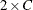or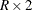. See the section Cochran-Armitage Test for Trend for details.

TSYMM
BOWKER

includes Bowker’s test of symmetry in the output data set. The AGREE option in the TABLES statement requests computation of Bowker’s test. See the section Bowker’s Test of Symmetry for details.

U

includes the uncertainty coefficient (symmetric) in the output data set. The MEASURES option in the TABLES statement requests computation of the uncertainty coefficient. See the section Uncertainty Coefficient (Symmetric) for details.

UCR

includes the asymmetric uncertainty coefficient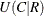in the output data set. The MEASURES option in the TABLES statement requests computation of the uncertainty coefficient. See the section Uncertainty Coefficients (Asymmetric) for details.

URC

includes the asymmetric uncertainty coefficient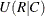in the output data set. The MEASURES option in the TABLES statement requests computation of the uncertainty coefficient. See the section Uncertainty Coefficients (Asymmetric) for details.

WTKAP
WTKAPPA

includes the weighted kappa coefficient in the output data set. The AGREE option in the TABLES statement requests computation of weighted kappa, which is available for square tables larger than. For multiway tables, the WTKAP option also includes the overall weighted kappa coefficient in the output data set. See the sections Weighted Kappa Coefficient and Overall Kappa Coefficient for details.